## Erik Cheever and Yue Li

### Abstract

This paper describes a program intended to be an aid for teaching and learning the process of creating Bode diagrams by hand. Bode diagrams are a fundamental tool for understanding the frequency domain behavior of systems. The program, BodePlotGui, is a GUI (graphical user interface) tool written in the MATLAB® programming language. This program takes as input a transfer function, splits it into its constituent elements, then draws the piecewise linear asymptotic approximation for each element.

### Introduction

Bode diagrams are critical to understanding frequency-domain analysis and design of linear systems. Although programs exist that accurately create Bode diagrams given a system transfer function (e.g., MATLAB®), students must still learn to draw Bode diagrams using piecewise-linear asymptotic approximations to understand fully their relationship to the transfer function. Drawing piecewise linear asymptotic Bode diagrams by hand is a process that is often difficult to learn (and to teach), but it is important for students’ understanding of how the locations of poles and zeros affect the shape of the graphs and, hence, the behavior of the system being studied.

Much of a student’s difficulty derives from the relative scarcity of examples. Typically, the only resources a student has are a few examples of in a textbook and perhaps a few from class notes. The ability to generate additional of asymptotic Bode diagrams examples to reinforce learned concepts, or to explore a range of “What if?” scenarios concerning pole and zero placement, would benefit many students. MATLAB does have a function to generate such plots, BodeAsym, but it has several shortcomings. Among the shortcomings: the function graphs only magnitude; it does not show the individual terms of the transfer function separately; and does not show resonant peaks in underdamped systems.

The difficulty in teaching Bode diagrams is twofold. First, there is the conceptual problem of the derivation of the asymptotic approximations and a description of just what the Bode diagrams represent. This paper does not address these issues. The second problem, however, is more mechanical and thus amenable to solution by computer; it is difficult to draw asymptotic Bode diagrams by hand. Drawing semi-logarithmic graphs by hand is inaccurate, whether it be on a chalkboard, whiteboard or on an overhead transparency. Paper handouts with examples worked out can be useful. They are not, however, generally useful for answering a student’s “What if?” questions, due to their static nature. Preprinted axes on overhead transparencies are another option, but these can be prone to errors, and also have difficulty answering many “What if?” questions before the transparencies become cluttered and unusable.

This paper describes a software tool designed to meliorate difficulties of students and of teachers. The tool is a GUI (Graphical User Interface) written in MATLAB that takes as input a system transfer function. The program separates the function into its constituent terms (i.e., poles, zeroes, and a constant term) and graphs the asymptotic approximation for each. The user can then choose to selectively exclude (or include) specific elements to simplify the transfer function or to isolate the behavior of a single part. The software is at http://www.swarthmore.edu/NatSci/echeeve1/Ref/Bode/BodeFiles.html.

### The Software

A description of the software follows, along with several examples. Due to the length of the code, close to one thousand lines of code comprising nine functions, only a brief outline of the software is given. The algorithm used  by the software to draw asymptotic Bode plots closely follows the rules taught to undergraduates.

The software is invoked via the MATLAB command BodePlotGui(Sys) where Sys is a transfer function object in the MATLAB environment. The first function called by the GUI is BodePlotTerms. This function takes as input a transfer function and decomposes it into seven different types of elements: a constant term, real poles and zeros (not at the origin), complex poles and zeros, and poles and zeros at the origin. The function then determines if any poles and zeros have a multiplicity greater than one by checking if they are equal within a predefined accuracy (set by default to 1 part in 10^3). As a last step, the function checks that all poles or zeros are in the left half of the s-plane (negative real part). If there are poles in the right half-plane, the program prints a warning and quits. If there are zeros in the right half-plane, it prints a warning that this is a non-minimum phase system, but continues. Poles and/or zeros on or very near the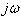axis (excluding the origin) result in a warning that the Bode plot is likely to be inaccurate near those frequencies (since the function is going to zero or infinity, which is impossible to show on a logarithmic, dB, scale).

The next function called is BodePlotter, and it does the bulk of the work. It takes each term (from BodePlotTerms) and draws a piecewise linear approximation for magnitude and phase. To these diagrams it adds the exact magnitude and phase functions (using the built in MATLAB function bode). Last, it displays the transfer function displayed by calling another function, BodePlotDispTF.

The rest of the functions are devoted to the operation of the GUI, and are not discussed here. An interested reader can refer to the MATLAB code.

### A Simple Example

Consider the transfer function: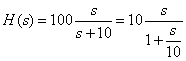This function has three separate terms to be considered when constructing a Bode diagram:

1. a multiplicative constant of 10 (20 dB),
2. a pole at ω=10 sec-1, and
3. a zero at the origin.

The following MATLAB commands begin execution of the GUI:

>> MySys=tf(100*[1 0],[1 10]); %define Xfer function
>> BodePlotGui(MySys) %Invoke GUI

The GUI generates a window as shown in Figure 1. Starting in the upper left and going clockwise, the windows show:

1. The magnitude plot, both the piecewise linear approximation for all three terms as well as the exact Bode diagram for magnitude. Also shown is a zero reference.
2. The phase plot.
3. A box that allows the user to format the plot by changing the width of the lines, adding grids...
4. The legend identifying individual terms on the plot.
5. A box that shows elements excluded from the plot. This box is empty in this display because the diagram displays all three elements of the transfer function.
6. A box that shows elements displayed in the plot.

Also shown in the upper right hand corner is the transfer function, H(s).

 Figure 1 Display of the GUI for a simple transfer function with a pole at ω=10 sec-1, a zero at the origin, and a constant term of 100.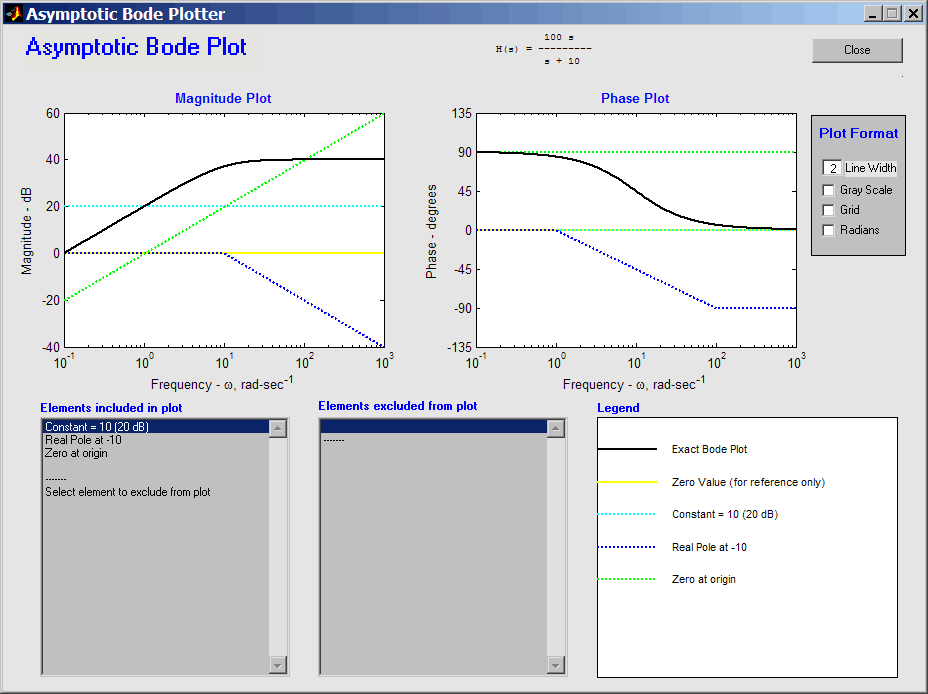Click here to view plot with the individual elements included or excluded from the plot.  (On the plot, check or Uncheck boxes to include or exclude elements from plot.)

The function displayed can be manipulated term by term to illustrate the effect of each term. For example, the zero at the origin can be excluded simply by clicking on it in the lower left hand box. Figure 2 shows the result, along with an annotation describing the operation performed. Note that the zero term is gone from the display and the transfer function, H(s), and legend have changed to reflect this.

 Figure 2 Identical to Figure 1, but with the zero at the origin excluded. Clicking on the term when it was in the Elements included window moved it to the Elements excluded window. The legend and transfer function (at top of window) have changed to reflect the change.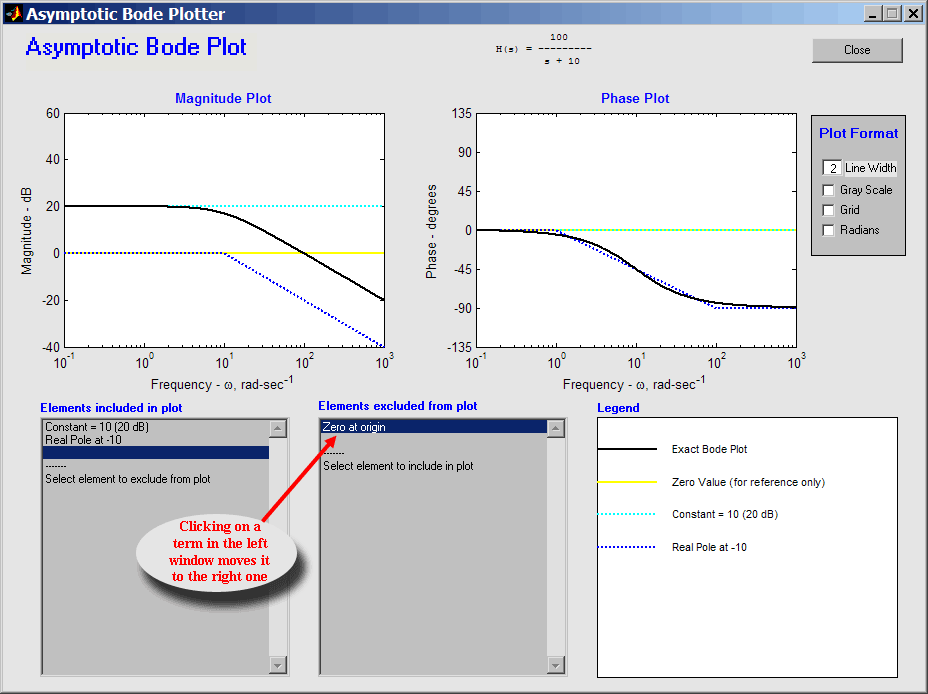### Complex Poles and Zeros

When constructing Bode diagrams underdamped systems, with complex poles and/or zeros present an added difficulty because of the resonant peaks. The software described here graphs the piecewise linear asymptotic Bode diagram and also shows the resonance that develops as a deviation from the zero decibel line. For an underdamped pole the peak height is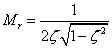and occurs at the frequency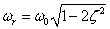These are well-known equations and occur in myriad books on Linear Systems or Control Theory .

Drawing the phase for a complex pole or zero is more complicated. There are no well-known rules for drawing piecewise linear approximations for the phase of underdamped systems. Instead, most textbooks use phrases like “There are no easy ways to draw such phase plots; refer to the phase-angle plots in Figure …” to guide students. Clearly, this advice is not helpful to the creation of a computer algorithm for drawing phase plots. To develop an approximation to use with this program the error between the actual phase and a variety of different piecewise linear functions was calculated for a wide range of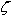. Empirically it was determined that, for an underdamped pole, a piecewise approximation that is 0º for low frequencies, -180º for high frequencies and linearly varying between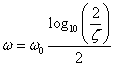and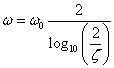yielded a small error between the approximation and the exact value of the phase. If<0.02 a straight line is drawn from 0º to -180º at ω=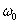.

Figure 3 shows an example of a system with an underdamped pole. In this figure the transfer function, H(s) is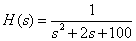This transfer function yields two separate terms for the Bode diagram:

1. a dc gain of 0.01 (-40 dB), and
2. a complex pole with=0.1 and=10.

Note that Figure 3 shows the resonant peak, and that the legend shows bothandas well as the location of the pole in the s-plane (i.e.,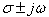).

 Figure 3 This diagram demonstrates the display of an underdamped (resonant) term. The magnitude plot shows a displacement from the 0 dB line as a single point. The piecewise linear phase approximation is described in the text. The legend gives the natural frequency and damping ratio as well as the pole location in the s-plane.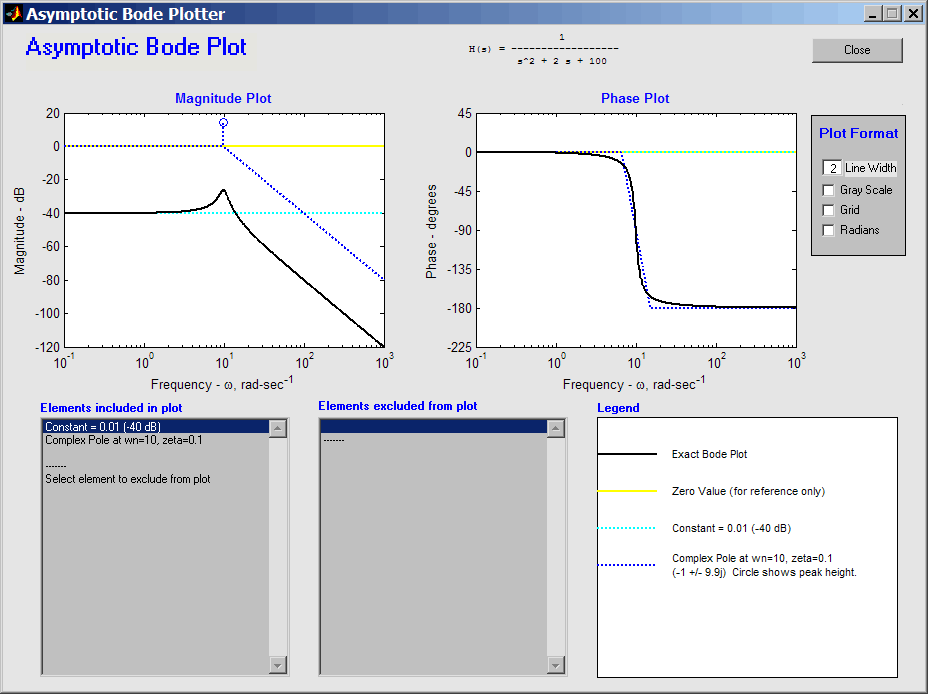Click here to view this plot with the individual elements included or excluded from the plot.  (On the plot, check or Uncheck boxes to include or exclude elements from plot.)

### A More Complicated Example

Now consider a more complicated transfer function with repeated poles. To wit, let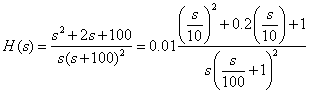This transfer function has 4 separate terms to be plotted:

1. a constant of 0.01=-40 dB,
2. a pole at the origin,
3. a repeated pole at ω=100, and
4. a complex zero with=0.1 and=10.

Figure 4 shows the output from the program. Note that the legend indicates that the pole at ω=100 has a multiplicity of two.

 Figure 4 When a pole (or zero) is repeated the GUI notes this and changes the display accordingly. The legend and Items included windows both show a multiplicity of two, and the magnitude and phase plots reflect the double pole at ω=100 sec-1.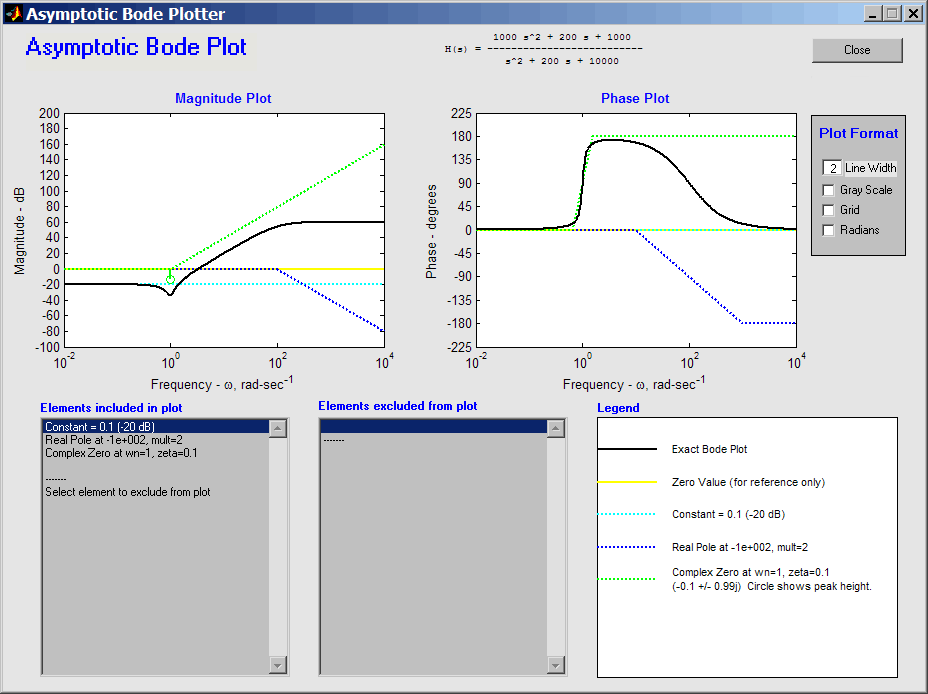Click here to view the plot with individual elements included or excluded from the plot.  (On the plot, check or Uncheck boxes to include or exclude elements from plot.)

### Limitations

The software has some known limitations. It does not handle the case of a time delay. It can only decompose transfer functions with up to 20 separate terms due to the number of colors used. Repeated poles and zeros are treated as a single term (i.e., it is not possible to move only one pole of a repeated pair from the “Elements included in plot” window to the “Elements excluded from plot” window of the GUI).

### Conclusions

The BodePlotGui program is a versatile program for teaching and learning the construction of Bode diagrams from piecewise linear approximations.

For teachers, it offers the ability to generate multiple examples in class with neat accurate drawings. Furthermore, it enables the teacher to isolate the individual elements of the Bode diagram to demonstrate how each contributes to the resultant plots.

For students, this software gives them the ability to generate multiple examples to help in the learning process. He or she can take examples from class or from a textbook and reproduce them. They can also break down any example they find, or create themselves, into its constituent parts. Moreover, they can do so at their own pace.

### References

1. K. Ogata, System Dynamics, 3rd edition, pp 480-481. Prentice Hall, Upper Saddle River, NJ (1998).

### Biographical Sketches

Erik Cheever is a professor of engineering at Swarthmore College. He received his BS in engineering from Swarthmore College and his Masters and PhD in Bioengineering from the University of Pennsylvania. Professor Cheever's teaching interests include circuits, linear systems and signal processing. His research interests include using embedded web servers for microcontroller user interfaces, and designing genetic algorithms for analog circuit design. He can be reached by email at erik_cheever@swarthmore.edu, his web page is http://www.swarthmore.edu/NatSci/echeeve1.

Yue Li is a senior student at Swarthmore College. He majors in Engineering and minors in Environmental Science. He received all his education in Shanghai, China before he came to Swarthmore three years ago. He has basic knowledge of CSS and Javascript and is involved in several web design projects at Swarthmore College. He can be reached by email at yli3@swarthmore.edu.